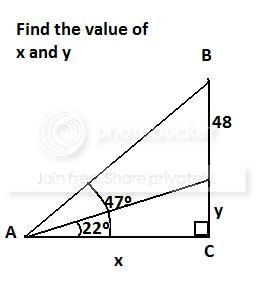1. I don't understand this problem...thanks.2.

3.Originally Posted by BitterSweet
I don't understand this problem...thanks.
This is quite obviously a homework problem. I you know what a tangent is you ought to be able to solve it.

If not it would behoove you to open your book and learn.4. i do know about sine, cosine, tangent secant etc.

tanA = 48+y / x
multiply x to both sides:
(x)tanA = 48+y/x (x)
(x)tanA = 48+y

and i get lost...5.Originally Posted by BitterSweet
i do know about sine, cosine, tangent secant etc.

tanA = 48+y / x
multiply x to both sides:
(x)tanA = 48+y (x)

and i get lost...

This is clearly a homework problem so no one is going to do the problem for you. You might want to review your algebra -- 2 equations and 2 unknowns.6. You posted one and only one relationship seen in your diagram. Try finding others. In general you need as many independent relationships as there are unknowns. You mentioned one equation with 2 unknowns.

Be more careful in writing your formulas.

By 1+2/3 do you mean (1+2)/3 or 1+(2/3)?Bookmarks
 Posting Permissions
 You may not post new threads You may not post replies You may not post attachments You may not edit your posts   BB code is On Smilies are On [IMG] code is On [VIDEO] code is On HTML code is Off Trackbacks are Off Pingbacks are Off Refbacks are On Terms of Use Agreement